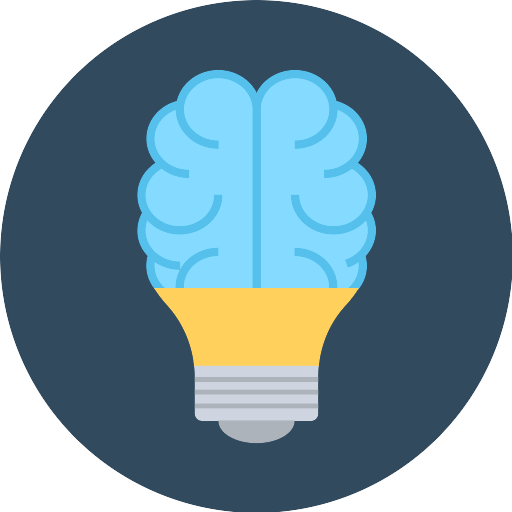k-Means clustering with Spark is easy to understand. MLlib comes bundled with k-Means implementation (KMeans) which can be imported from pyspark.mllib.clustering package. Here is a very simple example of clustering data with height and weight attributes.

Arguments to KMeans.train:

1. k is the number of desired clusters
2. maxIterations is the maximum number of iterations to run.
3. runs is the number of times to run the k-means algorithm
4. initializationMode can be either ‘random’or ‘k-meansII’
```from pyspark import SparkContext from pyspark.mllib.clustering import KMeans from numpy import array   sc = SparkContext() sc.setLogLevel ("ERROR")   #12 records with height, weight data data = array([185,72, 170,56, 168,60, 179,68, 182,72, 188,77, 180,71, 180,70, 183,84, 180,88, 180,67, 177,76]).reshape(12,2)   #Generate Kmeans model = KMeans.train(sc.parallelize(data), 2, runs=50, initializationMode="random")   #Print out the cluster of each data point print (model.predict(array([185, 71]))) print (model.predict(array([170, 56]))) print (model.predict(array([168, 60]))) print (model.predict(array([179, 68]))) print (model.predict(array([182, 72]))) print (model.predict(array([188, 77]))) print (model.predict(array([180, 71]))) print (model.predict(array([180, 70]))) print (model.predict(array([183, 84]))) print (model.predict(array([180, 88]))) print (model.predict(array([180, 67]))) print (model.predict(array([177, 76])))```

Output

```#10 items go to cluster 0, where as 2 items go to cluster 2
0
1
1
0
0
0
0
0
0
0
0
0
```

Above is a very naive example in which we use training dataset as input data too. In real world we will train a model, save it and later use it for predicting clusters of input data. So here is how you can save a trained model and later load it for prediction.

Training and Storing the Model

```from pyspark import SparkContext from pyspark.mllib.clustering import KMeans from numpy import array   sc = SparkContext()   #12 records with height, weight data data = array([185,72, 170,56, 168,60, 179,68, 182,72, 188,77, 180,71, 180,70, 183,84, 180,88, 180,67, 177,76]).reshape(12,2)   #Generate Kmeans model = KMeans.train(sc.parallelize(data), 2, runs=50, initializationMode="random")   model.save(sc, "savedModelDir")```

This will create a directory, savedModelDir with two subdirectories data and metadata where the model is stored.

Using Already Trained Model for Predicting Clusters
Now, let’s use trained model by loading it. We need to import KMeansModel in order to use it for loading the model from file.

```from pyspark import SparkContext from pyspark.mllib.clustering import KMeans, KMeansModel from numpy import array   sc = SparkContext()   #Generate Kmeans model = KMeansModel.load(sc, "savedModelDir")   #Print out the cluster of each data point print (model.predict(array([185, 71]))) print (model.predict(array([170, 56]))) print (model.predict(array([168, 60]))) print (model.predict(array([179, 68]))) print (model.predict(array([182, 72]))) print (model.predict(array([188, 77]))) print (model.predict(array([180, 71]))) print (model.predict(array([180, 70]))) print (model.predict(array([183, 84]))) print (model.predict(array([180, 88]))) print (model.predict(array([180, 67]))) print (model.predict(array([177, 76]))) (adsbygoogle=window.adsbygoogle||[]).push({}); ```

References:## Want to Learn More? Signup in a Click.